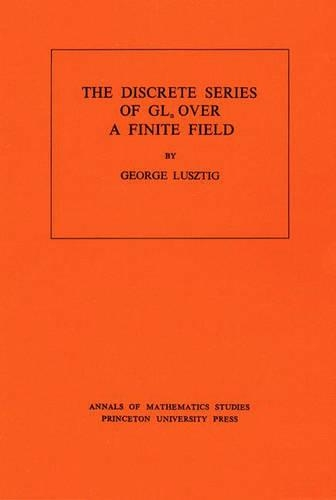•Discrete Series of GLn Over a Finite Field. (AM-81), Volume 81 - Annals of Mathematics Studies 81 (Paperback)

(author)
£49.00
Paperback 104 Pages / Published: 01/07/1992
• We can order this

Usually dispatched within 3 weeks

In this book Professor Lusztig solves an interesting problem by entirely new methods: specifically, the use of cohomology of buildings and related complexes. The book gives an explicit construction of one distinguished member, D(V), of the discrete series of GLn (Fq), where V is the n-dimensional F-vector space on which GLn(Fq) acts. This is a p-adic representation; more precisely D(V) is a free module of rank (q--1) (q2--1)...(qn-1--1) over the ring of Witt vectors WF of F. In Chapter 1 the author studies the homology of partially ordered sets, and proves some vanishing theorems for the homology of some partially ordered sets associated to geometric structures. Chapter 2 is a study of the representation ? of the affine group over a finite field. In Chapter 3 D(V) is defined, and its restriction to parabolic subgroups is determined. In Chapter 4 the author computes the character of D(V), and shows how to obtain other members of the discrete series by applying Galois automorphisms to D(V). Applications are in Chapter 5. As one of the main applications of his study the author gives a precise analysis of a Brauer lifting of the standard representation of GLn(Fq).

Publisher: Princeton University Press
ISBN: 9780691081540
Number of pages: 104
Weight: 170 g
Dimensions: 229 x 152 x 6 mm